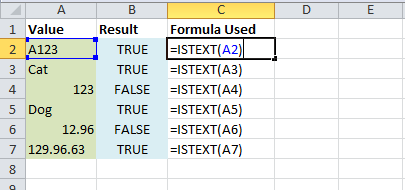This function will test if a value is text and return TRUE if it is text and FALSE if it’s not.

## Syntax

ISTEXT(Value)

• Value (required) – This is the value you want to test if it’s text.

## Example

In this example we test a few values. Notice that even though a value can contain numbers the result is still text.

``=ISTEXT(A2)``